# Data Structures, Algorithms, & Applications in Java Double-Ended Priority Queues Copyright 1999 Sartaj Sahni

Definition
Application to External Sorting
Generic Methods for DEPQs
Interval Heaps
Definition
Inserting an Element
Removing the Min Element
Initializing an Interval Heap
Complexity of Interval Heap Operations
The Complementary Range Search Problem

Definition
A double-ended priority queue (DEPQ) is a collection of zero or more elements. Each element has a priority or value. The operations performed on a double-ended priority queue are:
1. `isEmpty()` ... return true iff the DEPQ is empty
2. `size()` ... return the number of elements in the DEPQ
3. `getMin()` ... return element with minimum priority
4. `getMax()` ... return element with maximum priority
5. `put(x)` ... insert the element `x` into the DEPQ
6. `removeMin()` ... remove an element with minimum priority and return this element
7. `removeMax()` ... remove an element with maximum priority and return this element

Application to External Sorting
The internal sorting method that has the best expected run time is quick sort (see Section 19.2.3). The basic idea in quick sort is to partition the elements to be sorted into three groups `L`, `M`, and `R`. The middle group `M` contains a single element called the pivot, all elements in the left group `L` are `<=` the pivot, and all elements in the right group `R` are `>=` the pivot. Following this partitioning, the left and right element groups are sorted recursively.

In an external sort, we have more elements than can be held in the memory of our computer. The elements to be sorted are initially on a disk and the sorted sequence is to be left on the disk. When the internal quick sort method outlined above is extended to an external quick sort, the middle group `M` is made as large as possible through the use of a DEPQ. The external quick sort strategy is:
1. Read in as many elements as will fit into an internal DEPQ. The elements in the DEPQ will eventually be the middle group of elements.
2. Read in the remaining elements. If the next element is `<=` the smallest element in the DEPQ, output this next element as part of the left group. If the next element is `>=` the largest element in the DEPQ, output this next element as part of the right group. Otherwise, remove either the max or min element from the DEPQ (the choice may be made randomly or alternately); if the max element is removed, output it as part of the right group; otherwise, output the removed element as part of the left group; insert the newly input element into the DEPQ.
3. Output the elements in the DEPQ, in sorted order, as the middle group.
4. Sort the left and right groups recursively.

Generic Methods for DEPQs
General methods exist to arrive at efficient DEPQ data structures from single-ended priority queue (PQ) data structures that also provide an efficient implementation of the `remove(theNode)` operation (this operation removes the node `theNode` from the PQ). The simplest of these methods, dual structure method, maintains both a min PQ and a max PQ of all the DEPQ elements together with correspondence pointers between the nodes of the min PQ and the max PQ that contain the same element. Figure 1 shows a dual heap structure for the elements ```6, 7, 2, 5, 4```. Correspondence pointers are shown as red arrows.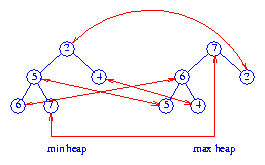Figure 1 Dual heap

Although the figure shows each element stored in both the min and the max heap, it is necessary to store each element in only one of the two heaps.

The `isEmpty` and `size` operations are implemented by using a variable `size` that keeps track of the number of elements in the DEPQ. The minimum element is at the root of the min heap and the maximum element is at the root of the max heap. To insert an element `x`, we insert `x` into both the min and the max heaps and then set up correspondence pointers between the locations of `x` in the min and max heaps. To remove the minimum element, we do a `removeMin` from the min heap and a `remove(theNode)`, where `theNode` is the corresponding node for the removed element, from the max heap. The maximum element is removed in an analogous way.

Total and leaf correspondence are more sophisticated correspondence methods. In both of these, half the elements are in the min PQ and the other half in the max PQ. When the number of elements is odd, one element is retained in a buffer. This buffered element is not in either PQ. In total correspondence, each element `a` in the min PQ is paired with a distinct element `b` of the max PQ. `(a,b)` is a corresponding pair of elements such that `priority(a) <= priority(b)`. Figure 2 shows a total correspondence heap for the `11` elements `2, 3, 4, 4, 5, 5, 6, 7, 8, 9, 10`. The element `9` is in the buffer. Corresponding pairs are shown by red arrows.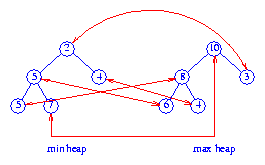Figure 2 Total correspondence heap

In leaf correspondence, each leaf element of the min and max PQ is required to be part of a corresponding pair. Nonleaf elements need not be in any corresponding pair. Figure 3 shows a leaf correspondence heap.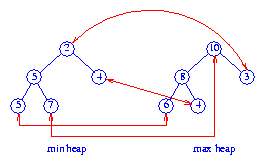Figure 3 A leaf correspondence heap

Total and leaf correspondence structures require less space than do dual structures. However, the DEPQ algorithms for total and leaf correspondence structures are more complex than those for dual structures. Of the three correspondence types, leaf correspondence generally results in the fastest DEPQ correspondence structures.

Using any of the described correspondence methods, we can arrive at DEPQ structures from heaps, height biased leftist trees, and pairing heaps. In these DEQP structures, the operations `put(x)`, `removeMin()`, and `removeMax()` take `O(log n)` time (`n` is the number of elements in the DEPQ, for pairing heaps, this is an amortized complexity), and the remaining DEPQ operations take `O(1)` time.

Interval Heaps
Definition
Although the idea of correspondence results in generic strategies to obtain an efficient DEPQ structure from an efficient PQ structure that also supports the `remove(theNode)` operation, we can, at times, obtain more elegant and more efficient DEPQ structures using a custom strategy. This, for example, is the case when adapting the heap structure. For the heap structure, many adaptation strategies are possible. These strategies have resulted in the DEPQ structures min-max heaps, twin heaps, deaps, diamond deque, and interval heaps. The simplest and most efficient of these is the interval heap.

An interval heap is a complete binary tree in which each node, except possibly the last one (the nodes of the complete binary tree are ordered using a level order traversal), contains two elements. Let the priorities of the two elements (in the sequel, we do not differentiate between an element and its priority) in node `P` be `a` and `b`, where `a <= b`. We say that the node `P` represents the closed interval `[a,b]`. `a` is the left end point of the interval of `P`, and `b` is its right end point. The interval `[c,d]` is contained in the interval `[a,b]` iff `a <= c <= d <= b`. In an interval heap, the intervals represented by the left and right children (if they exist) of each node `P` are contained in the interval represented by `P`. When the last node contains a single element with priority `c`, then `a <= c <= b`, where `[a,b]` is the interval of the parent (if any) of the last node.

Figure 4 shows an interval heap with `26` elements, only the element priorities are shown. You may verify that the intervals represented by the children of any node `P` are contained in the interval of `P`.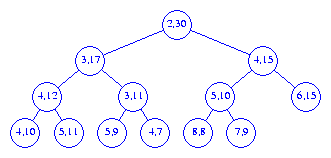Figure 4 An interval heap

The following facts are immediate:
1. The left end points of the node intervals define a min heap, and the right end points define a max heap. In case the number of elements is odd, the last node has a single element which may be regarded as a member of either the min or max heap. Figure 5 shows the min and max heaps defined by the interval heap of Figure 4.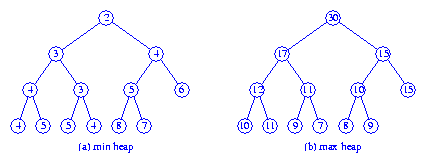Figure 5 Min and max heaps embedded in Figure 4

2. When the root has two elements, the left end point of the root is the minimum priority in the interval heap and the right end point is the maximum priority. When the root has only one element, the interval heap contains just one element. This element is both the minimum and maximum element.
3. An interval heap can be represented compactly by mapping into an array as is done for ordinary heaps. However, now, each array position must have space for two elements.
4. The height of an interval heap with `n` elements is `Theta(log n)`.

Inserting an Element
Suppose we are to insert an element into the interval heap of Figure 4. Since this heap currently has an even number of elements, the heap following the insertion will have an additional node `A` as is shown in Figure 6.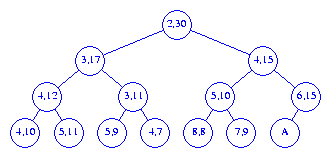Figure 6 Interval heap of Figure 4 after one node is added

The interval for the parent of the new node `A` is `[6,15]`. Therefore, if the priority of the new element is between `6` and `15`, the new element may be inserted into node `A`. When the priority of the new element is less than the left end point `6` of the parent interval, the new element is inserted into the min heap embedded in the interval heap. This insertion is done using the min heap insertion procedure starting at node `A`. When the priority of the new element is greater than the right end point `15` of the parent interval, the new element is inserted into the max heap embedded in the interval heap. This insertion is done using the max heap insertion procedure starting at node `A`.

If we are to insert an element with priority `10` into the interval heap of Figure 4, this element is put into the node `A` shown in Figure 6. To insert an element with priority `3`, we follow a path from node `A` towards the root, moving left end points down until we either pass the root or reach a node whose left end point is ```<= 3```. The new element is inserted into the node that now has no left end point. Figure 7 shows the resulting interval heap.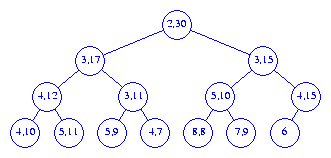Figure 7 The interval heap of Figure 4 with 3 inserted

To insert an element with priority `40` into the interval heap of Figure 4, we follow a path from node `A` (see Figure 6) towards the root, moving right end points down until we either pass the root or reach a node whose right end point is ```>= 40```. The new element is inserted into the node that now has no right end point. Figure 8 shows the resulting interval heap.Figure 8 The interval heap of Figure 4 with 40 inserted

Now, suppose we wish to insert an element into the interval heap of Figure 8. Since this interval heap has an odd number of elements, the insertion of the new element does not increase the number of nodes. The insertion procedure is the same as for the case when we initially have an even number of elements. Let `A` denote the last node in the heap. If the priority of the new element lies within the interval `[6,15]` of the parent of `A`, then the new element is inserted into node `A` (the new element becomes the left end point of `A` if its priority is less than that of the element currently in `A`). If the priority of the new element is less than the left end point `6` of the parent of `A`, then the new element is inserted into the embedded min heap; otherwise, the new element is inserted into the embedded max heap. Figure 9 shows the result of inserting an element with priority `32` into the interval heap of Figure 8.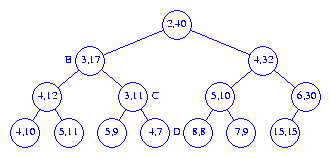Figure 9 The interval heap of Figure 8 with 32 inserted

Removing the Min Element
The removal of the minimum element is handled as several cases:
1. When the interval heap is empty, the `removeMin` operation fails.
2. When the interval heap has only one element, this element is the element to be returned. We leave behind an empty interval heap.
3. When there is more than one element, the left end point of the root is to be returned. This point is removed from the root. If the root is the last node of the interval heap, nothing more is to be done. When the last node is not the root node, we remove the left point `p` from the last node. If this causes the last node to become empty, the last node is no longer part of the heap. The point `p` removed from the last node is reinserted into the embedded min heap by beginning at the root. As we move down, it may be necessary to swap the current `p` with the right end point `r` of the node being examined to ensure that `p <= r`. The reinsertion is done using the same strategy as used to reinsert into an ordinary heap (see Program 13.3 of the text).

Let us remove the minimum element from the interval heap of Figure 9. First, the element with priority `2` is removed from the root. Next, the left end point `15` is removed from the last node and we begin the reinsertion procedure at the root. The smaller of the min heap elements that are the children of the root is `3`. Since this element is smaller than `15`, we move the `3` into the root (the `3` becomes the left end point of the root) and position ourselves at the left child `B` of the root. Since, `15 <= 17` we do not swap the right end point of `B` with the current `p = 15`. The smaller of the left end points of the children of `B` is `3`. The `3` is moved from node `C` into node `B` as its left end point and we position ourselves at node `C`. Since `p = 15 > 11`, we swap the two and `15` becomes the right end point of node `C`. The smaller of left end points of `C`s children is `4`. Since this is smaller than the current `p = 11`, it is moved into node `C` as this node's left end point. We now position ourselves at node `D`. First, we swap ```p = 11``` and `D`s right end point. Now, since `D` has no children, the current ```p = 7``` is inserted into node `D` as `D`s left end point. Figure 10 shows the result.Figure 10 The interval heap of Figure 9 with minimum element removed

The max element may removed using an analogous procedure.

Initializing an Interval Heap
Interval heaps may be initialized using a strategy similar to that used to initialize ordinary heaps--work your way from the heap bottom to the root ensuring that each subtree is an interval heap. For each subtree, first order the elements in the root; then reinsert the left end point of this subtree's root using the reinsertion strategy used for the `removeMin` operation, then reinsert the right end point of this subtree's root using the strategy used for the `removeMax` operation.

Complexity of Interval Heap Operations
The operations `isEmpty()`, `size()`, `getMin()`, and `getMax()` take `O(1)` time each; `put(x)`, `removeMin()`, and `removeMax()` take `O(log n)` each; and initializing an `n` element interval heap takes `O(n)` time.

The Complementary Range Search Problem
In the complementary range search problem, we have a dynamic collection (i.e., points are added and removed from the collection as time goes on) of one-dimensional points (i.e., points have only an x-coordinate associated with them) and we are to answer queries of the form: what are the points outside of the interval `[a,b]`? For example, if the point collection is ```3, 4, 5, 6, 8, 12```, the points outside the range `[5,7]` are `3, 4, 8, 12`.

When an interval heap is used to represent the point collection, a new point can be inserted or an old one removed in `O(log n)` time, where `n` is the number of points in the collection. Note that given the location of an aribtrary element in an interval heap, this element can be removed from the interval heap in `O(log n)` time using an algorithm similar to that used to remove an arbitrary element from a heap (see Exercise 13.13 of the text).

The complementary range query can be answered in `Theta(k)` time, where `k` is the number of points outside the range `[a,b]`. This is done using the following recursive procedure:
1. If the interval tree is empty, `return`.
2. If the root interval is contained in `[a,b]`, then all points are in the range (therefore, there are no points to report), `return`.
3. Report the end points of the root interval that are not in the range `[a,b]`.
4. Recursively search the left subtree of the root for additional points that are not in the range `[a,b]`.
5. Recursively search the right subtree of the root for additional points that are not in the range `[a,b]`.
6. `return`

Let us try this procedure on the interval heap of Figure 9. The query interval is `[4,32]`. We start at the root. Since the root interval is not contained in the query interval, we reach step 3 of the procedure. Whenever step 3 is reached, we are assured that at least one of the end points of the root interval is outside the query interval. Therefore, each time step 3 is reached, at least one point is reported. In our example, both points `2` and `40` are outside the query interval and so are reported. We then search the left and right subtrees of the root for additional points. When the left subtree is searched, we again determine that the root interval is not contained in the query interval. This time only one of the root interval points (i.e., `3`) is to be outside the query range. This point is reported and we proceed to search the left and right subtrees of `B` for additional points outside the query range. Since the interval of the left child of `B` is contained in the query range, the left subtree of `B` contains no points outside the query range. We do not explore the left subtree of `B` further. When the right subtree of `B` is searched, we report the left end point `3` of node `C` and proceed to search the left and right subtrees of `C`. Since the intervals of the roots of each of these subtrees is contained in the query interval, these subtrees are not explored further. Finally, we examine the root of the right subtree of the overall tree root, that is the node with interval `[4,32]`. Since this node's interval is contained in the query interval, the right subtree of the overall tree is not searched further.

The complexity of the above six step procedure is ```Theta(number of interval heap nodes visited)```. The nodes visited in the preceding example are the root and its two children, node `B` and its two children, and node `C` and its two children. Thus, `7` nodes are visited and a total of `4` points are reported.

We show that the total number of interval heap nodes visited is at most `3k + 1`, where `k` is the number of points reported. If a visited node reports one or two points, give the node a count of one. If a visited node reports no points, give it a count of zero and add one to the count of its parent (unless the node is the root and so has no parent). The number of nodes with a nonzero count is at most `k`. Since no node has a count more than `3`, the sum of the counts is at most `3k`. Accounting for the possibility that the root reports no point, we see that the number of nodes visited is at most `3k+1`. Therefore, the complexity of the search is `Theta(k)`. This complexity is asymptotically optimal because every algorithm that reports `k` points must spend at least `Theta(1)` time per reported point.

In our example search, the root gets a count of `2` (`1` because it is visited and reports at least one point and another `1` because its right child is visited but reports no point), node `B` gets a count of `2` (`1` because it is visited and reports at least one point and another `1` because its left child is visited but reports no point), and node `C` gets a count of `3` (`1` because it is visited and reports at least one point and another `2` because its left and right children are visited and neither reports a point). The count for each of the remaining nodes in the interval heap is `0`.